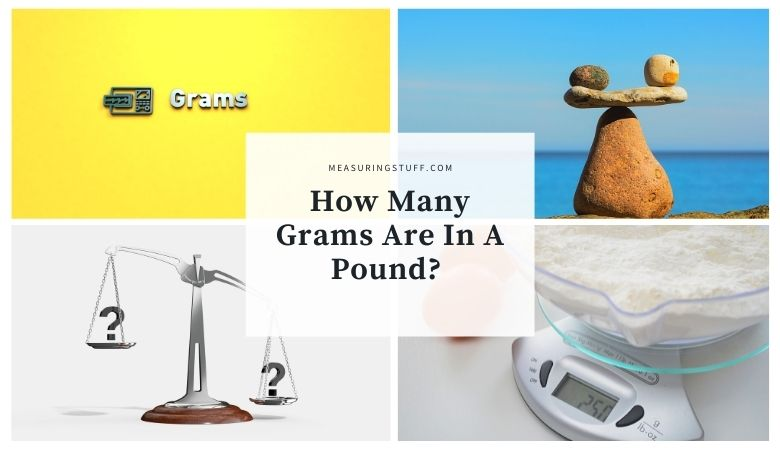# How Many Grams Are In A Pound?

Grams and pounds are both units used to measure weight. Although the two units are similar, they’re not the same, and converting between the two can be a bit tricky.

Luckily, a simple equation can help you easily figure out how many grams are in a pound or vice versa.

Grams are smaller than pounds, and 1 gram is equal to about .0022 pounds.

This means that, in order to figure out how many grams are in a certain number of pounds, you would divide the number of grams by 454.

For example, if you want to know how many pounds there are in 5,000 grams, you would divide 5,000 by 454.

5000 / 454 = 11

The answer, which is just over 11, tells you that there are about 11 pounds in 5,000 grams.

If you want to reverse the equation and find out how many grams there are in a certain number of pounds, you would multiply the number of pounds by 454 instead.

For example, if you have 10 pounds, you can multiply 10 by 454 to find that there are about 4,540 grams in 10 pounds.

10 x 454 = 4540

For even more accurate answers, you can also always use a conversion chart that calculates how many grams are in a pound or how many pounds can be made from a certain number of grams.

## Grams and Pounds Measurement

Grams are part of the metric system, which was created in France and is now the standard measurement system for most of the world.

Pounds, on the other hand, are part of the imperial system, which is mostly used in the United States.

## Pounds To Grams Conversion Calculator

Use the following calculator to convert pounds to grams and vice versa.

For our complete section of unit conversions including tables and calculators click the link here.